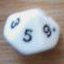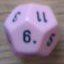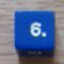Open-ended 2d6 result (dominions 4 game)

## Open-ended 2d6 result (dominions 4 game)

 Thu Oct 31 11:09:03 2013   by   Anon Hello,I can't find solution to calculate this kind of die roll:----This is a roll of two six-sided dice (2d6) but with an additional bonus: if any individual die roll is “6,” one is subtracted, and then that die is re-rolled and added to the result. This is referred to as an “open-ended” 2d6 roll.Example:  Two dice are rolled and they come up 2,6. Because one of thedice was a “6,” one is subtracted from the total (making 7), and the die is rolled again. But this die isalso a 6. So one is subtracted from the total (now up to 12) and a die is rolled again. It is a 4. The finalresult for this DRN is 16.Note that if both original dice came up as 6, both would be re-rolled and added as above. If a die keepscoming up 6, it keeps getting re-rolled and added, which can very occasionally lead to large numbers.-----Can someone help there?Thank you Fri Nov 1 10:03:18 2013   by   Torben The easiest is to treat each die separately and then add them at the end.  And it is also easier to roll the dice as z5's (dice with values from 0 to 5) and then add two to the result at the end.  So this should work:`2 + sum 2#(accumulate x := z5 while x=5)`